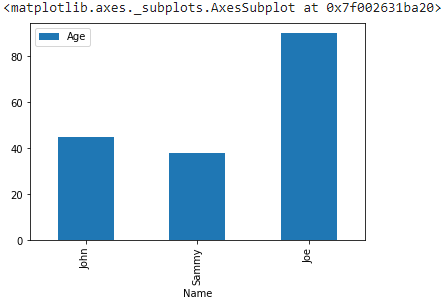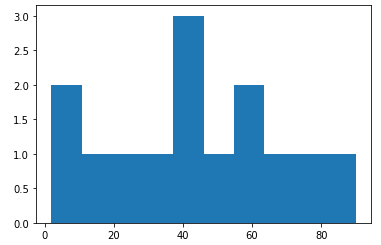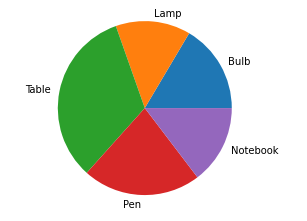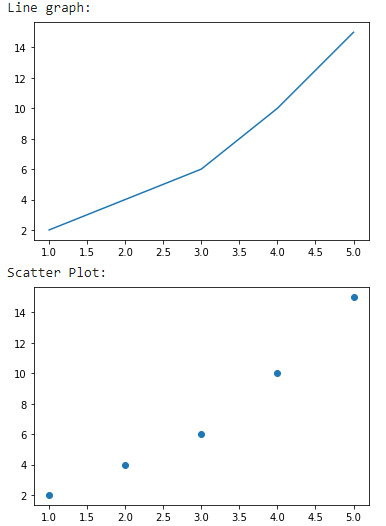# How to plot a Pandas Dataframe with Matplotlib?

• Last Updated : 24 Jan, 2021

Prerequisites:

Data visualization is the most important part of any analysis. Matplotlib is an amazing python library which can be used to plot pandas dataframe. There are various ways in which a plot can be generated depending upon the requirement.

## Comparison between categorical data

Bar Plot is one such example. To plot a bar graph using plot() function will be used.

Syntax:

matplotlib.pyplot.plot(\*args, scalex=True, scaley=True, data=None, \*\*kwargs)

Example:

## Python3

 `# importing pandas library ` `import` `pandas as pd ` `# importing matplotlib library ` `import` `matplotlib.pyplot as plt ` ` `  `# creating dataframe ` `df ``=` `pd.DataFrame({ ` `    ``'Name'``: [``'John'``, ``'Sammy'``, ``'Joe'``], ` `    ``'Age'``: [``45``, ``38``, ``90``] ` `}) ` ` `  `# plotting a bar graph ` `df.plot(x``=``"Name"``, y``=``"Age"``, kind``=``"bar"``) `

Output:## Visualizing continuous data

Histogram is an example of representing data as which is divided into closely related intervals. For this hist() function will be employed.

Syntax:

matplotlib.pyplot.hist(x, bins=None, range=None, density=False, weights=None, cumulative=False, bottom=None, histtype=’bar’, align=’mid’, orientation=’vertical’, rwidth=None, log=False, color=None, label=None, stacked=False, \*, data=None, \*\*kwargs)

Example:

## Python3

 `# importing pandas library ` `import` `pandas as pd ` `# importing matplotlib library ` `import` `matplotlib.pyplot as plt ` ` `  `# creating dataframe ` `df ``=` `pd.DataFrame({ ` `    ``'Age'``: [``45``, ``38``, ``90``, ``60``, ``40``, ``50``, ``2``, ``32``, ``8``, ``15``, ``27``, ``69``, ``73``, ``55``] ` `}) ` ` `  `# plotting a histogram ` `plt.hist(df[``"Age"``]) ` `plt.show() `

Output:## For data distribution

Pie Chart is a great way of representing data which is a part of a whole. To plot a pie chart pie() function will be used.

Syntax:

matplotlib.pyplot.pie(data, explode=None, labels=None, colors=None, autopct=None, shadow=False)

Example:

## Python3

 `# importing pandas library ` `import` `pandas as pd ` `# importing matplotlib library ` `import` `matplotlib.pyplot as plt ` ` `  `# creating dataframe ` `df ``=` `pd.DataFrame({ ` `    ``'Object'``: [``'Bulb'``, ``'Lamp'``, ``'Table'``, ``'Pen'``, ``'Notebook'``], ` `    ``'Price'``: [``45``, ``38``, ``90``, ``60``, ``40``] ` `}) ` ` `  `# plotting a pie chart ` `plt.pie(df[``"Price"``], labels``=``df[``"Object"``]) ` `plt.show() `

Output:## Dependency of data

In situations, where data is to be interpreted depending on dependent and non-dependent parameters, graphs like Line chart or Scatter plot, are used. To plot a line graph plot() function is sufficient but to visualize a scatter plot scatter() is used.

Syntax:

matplotlib.pyplot.scatter(x_axis_data, y_axis_data, s=None, c=None, marker=None, cmap=None, vmin=None, vmax=None, alpha=None, linewidths=None, edgecolors=None)

Example:

## Python3

 `# importing pandas library ` `import` `pandas as pd ` `# importing matplotlib library ` `import` `matplotlib.pyplot as plt ` ` `  `# creating dataframe ` `df ``=` `pd.DataFrame({ ` `    ``'X'``: [``1``, ``2``, ``3``, ``4``, ``5``], ` `    ``'Y'``: [``2``, ``4``, ``6``, ``10``, ``15``] ` `}) ` ` `  `# plotting a line graph ` `print``(``"Line graph: "``) ` `plt.plot(df[``"X"``], df[``"Y"``]) ` `plt.show() ` ` `  `# plotting a scatter plot ` `print``(``"Scatter Plot:  "``) ` `plt.scatter(df[``"X"``], df[``"Y"``]) ` `plt.show() `

Output:My Personal Notes arrow_drop_up
Recommended Articles
Page :